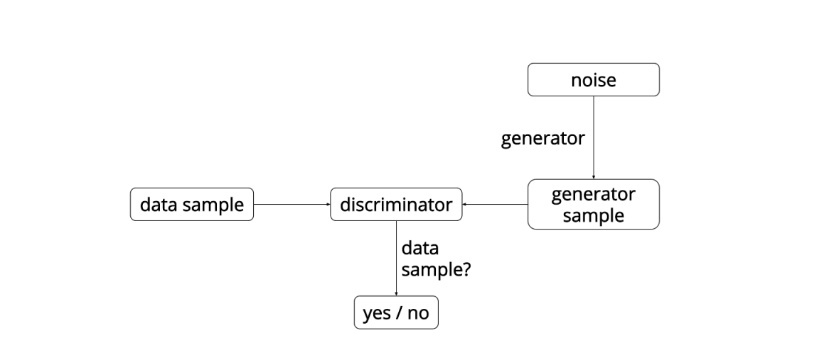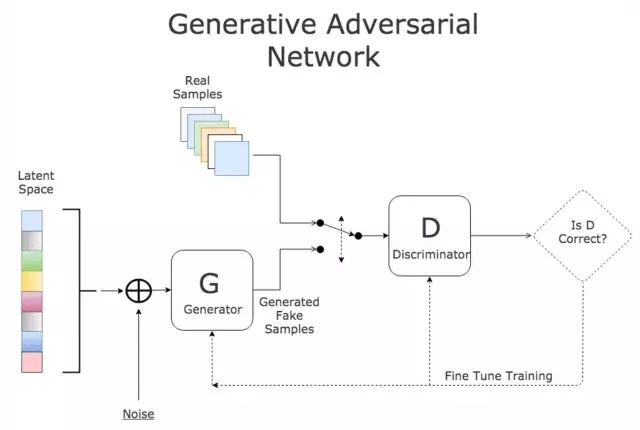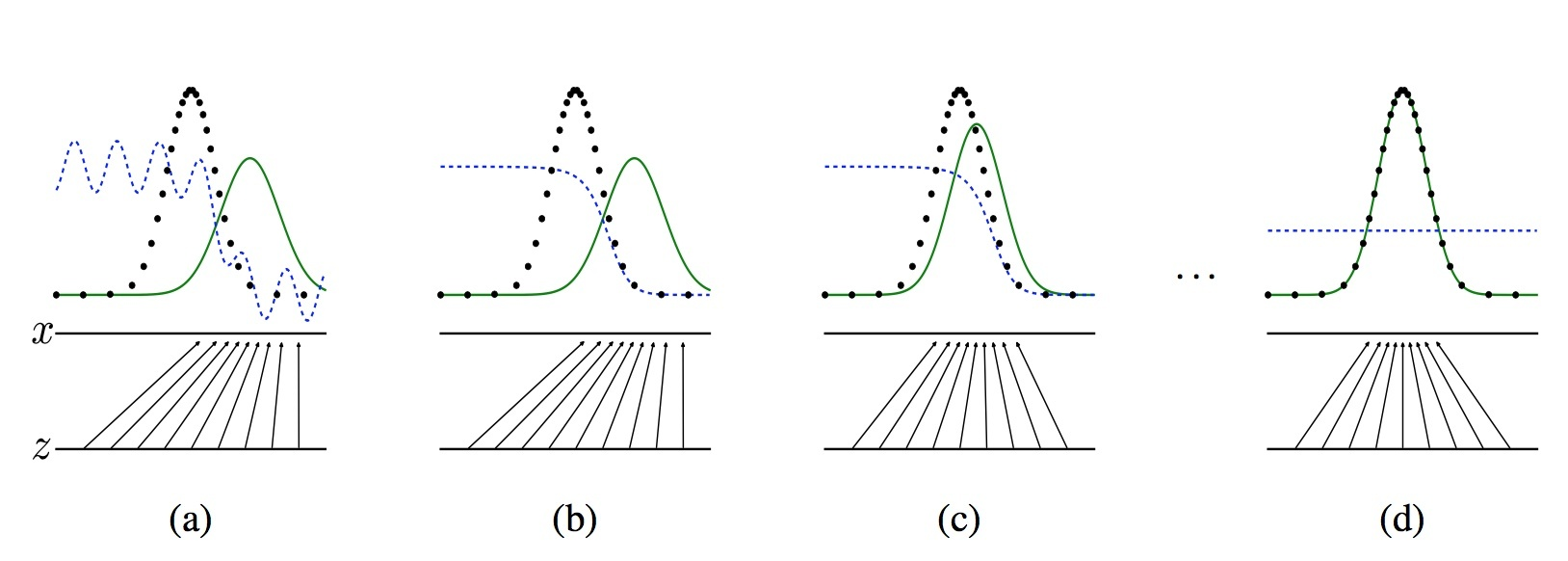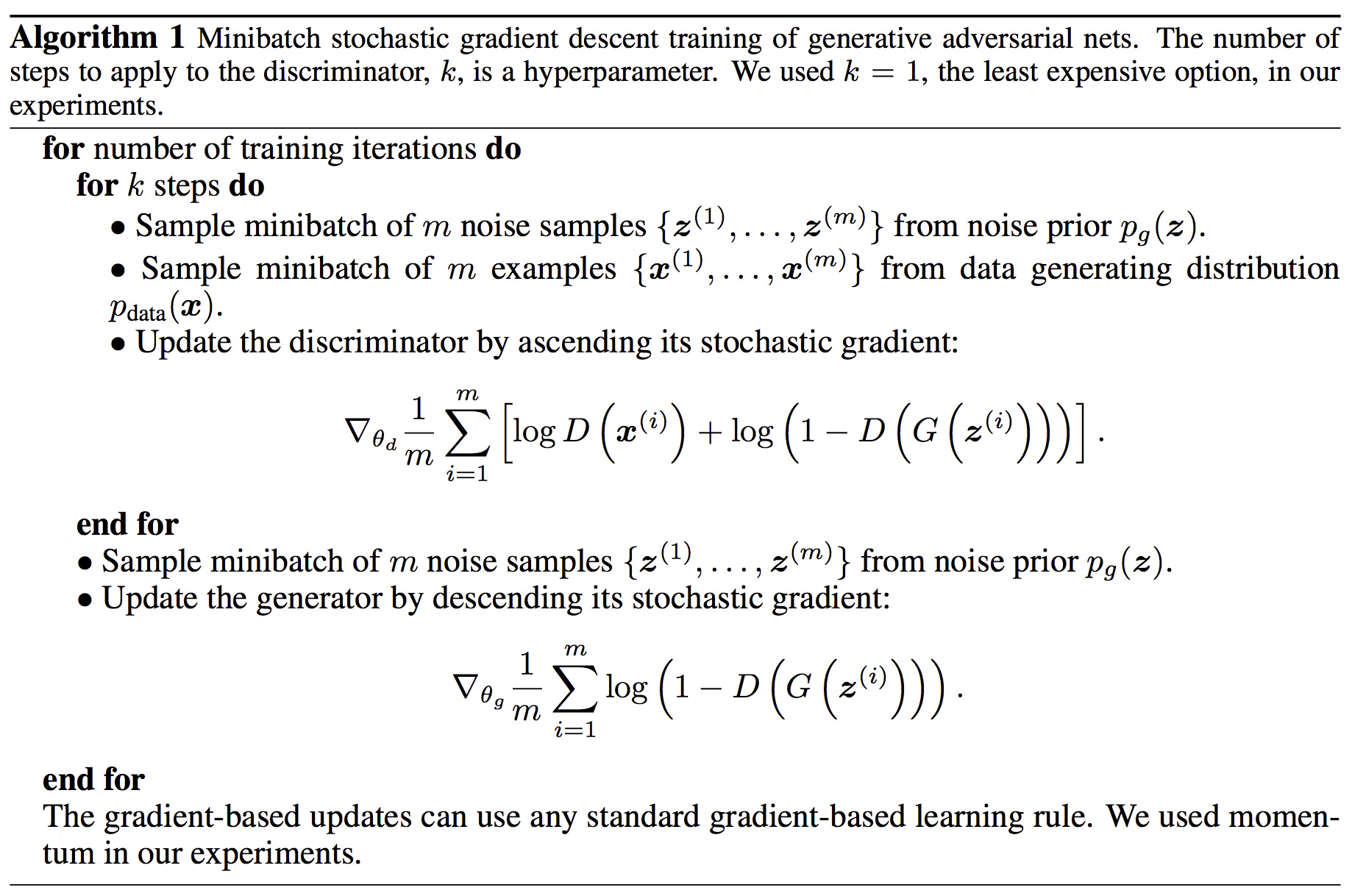## 概述

GAN是Ian Goodfellow提出的使用对抗过程来获得生成模型的新框架。

• 生成器 $$G$$ (Generator)
• 判别器 $$D$$ (discriminator)

1. 对于从训练数据中取样出的真实图片x，判别器D希望D(x)的输出值接近1，即判定训练数据为真实图片。

2. 给定一个随机噪声z，判别器D希望 $$D(G(z))$$ 的输出值接近 $$0$$ ，即认定生成器G生成的图片是假的；而生成器G希望 $$D(G(z))$$ 的输出值接近1，即G希望能够欺骗D，让D将生成器G生成的样本误判为真实图片。这样 G 和 D 就构成了博弈的状态。

3. 在博弈的过程中，生成器G和判别器D都不断的提升自己的能力，最后达到一个平衡的状态。G可以生成足以“以假乱真”的图片G(z)。对于D来说，它难以判定G生成的图片究竟是不是真实的，因此 $$D(G(z)) = 0.5$$ 。这样我们的目的就达成了：我们得到了一个生成式的模型G，它可以用来生成真实图片。• 对于图a，D还刚开始训练，本身分类的能力还很有限，有波动，但是初步区分实际数据和生成数据还是可以的。
• 图b，D训练得比较好了，可以很明显的区分出生成数据。
• 图c：绿色的线与黑色的线的偏移，蓝色的线下降了，也就是生成数据的概率下降了。那么，由于绿色的线的目标是提升概率，因此就会往蓝色线高的方向移动。那么随着训练的持续，由于G网络的提升，G也反过来影响D的分布。假设固定G网络不动，训练D，那么训练到最优， $$D^*_g(x) = p_{data}(x)/(p_{data}(x)+p_{g}(x))$$ 。
• 因此，随着 $$p_g(x)$$ 趋近于 $$p_{data}(x),D^*_g(x)$$ 会趋近于 $$0.5$$ ，也就是到图d。而我们的目标就是希望绿色的线能够趋近于黑色的线，也就是让生成的数据分布与实际分布相同。图d符合我们最终想要的训练结果。到这里，G网络和D网络就处于纳什均衡状态，无法再进一步更新了。

GAN 模型的目标函数：

$$\min_{G} \max_D V(G,D)=E_{x\sim P_{data}}[logD(x)] + E_{x\sim P_G}[log(1-D(x))]$$

G网络的loss是 $$log(1-D(G(z))$$ ，

### 训练

1. 固定G时，训练D；
$$\max {E_{x\sim p(r)} log(D(x)) + E_{x\sim p(g)} log(1-D(x)) }$$（公式1）
转化成最小形式：
$$\min -[{E_{x\sim p(r)} log(D(x)) + E_{x\sim p(g)} log(1-D(x)) } ]$$
2. 固定D时，训练G。
可以设置超参数k, 表示训练k次D，再训练一次G.
$$min Loss_G =E_{x\sim P_G}[log(1-D(x))]$$（公式2）